Ex 4.2

Chapter 4 Class 12 Determinants
Serial order wise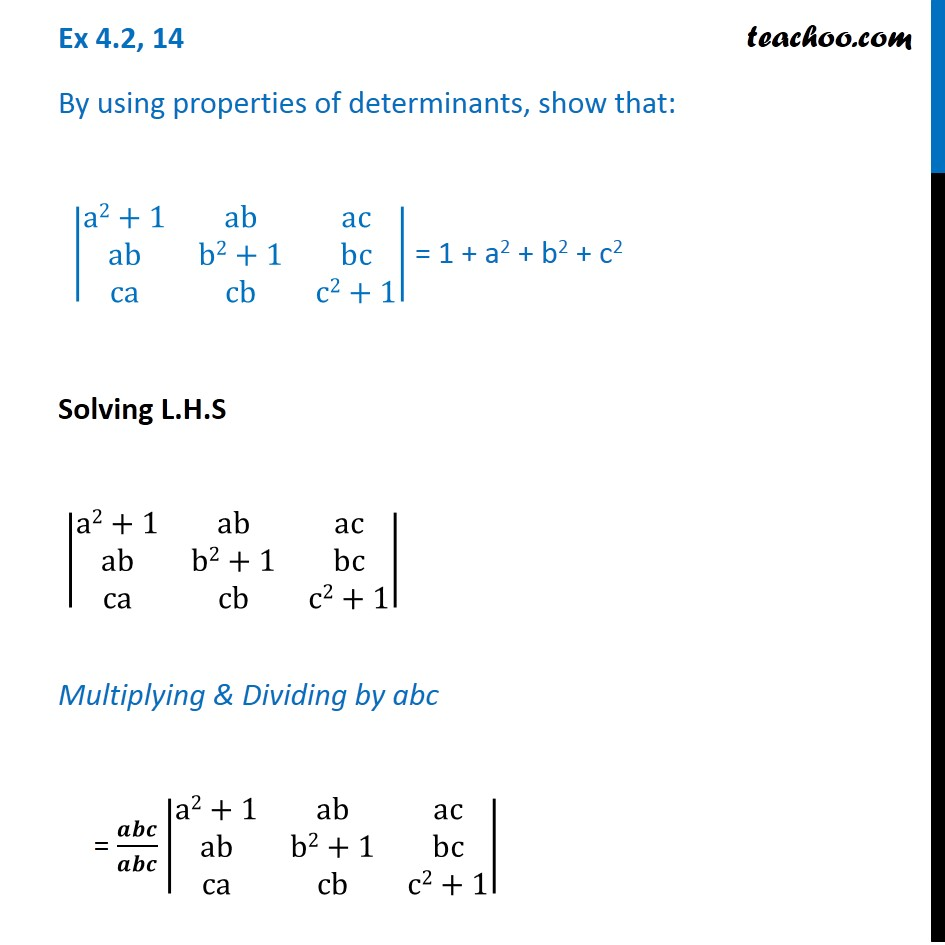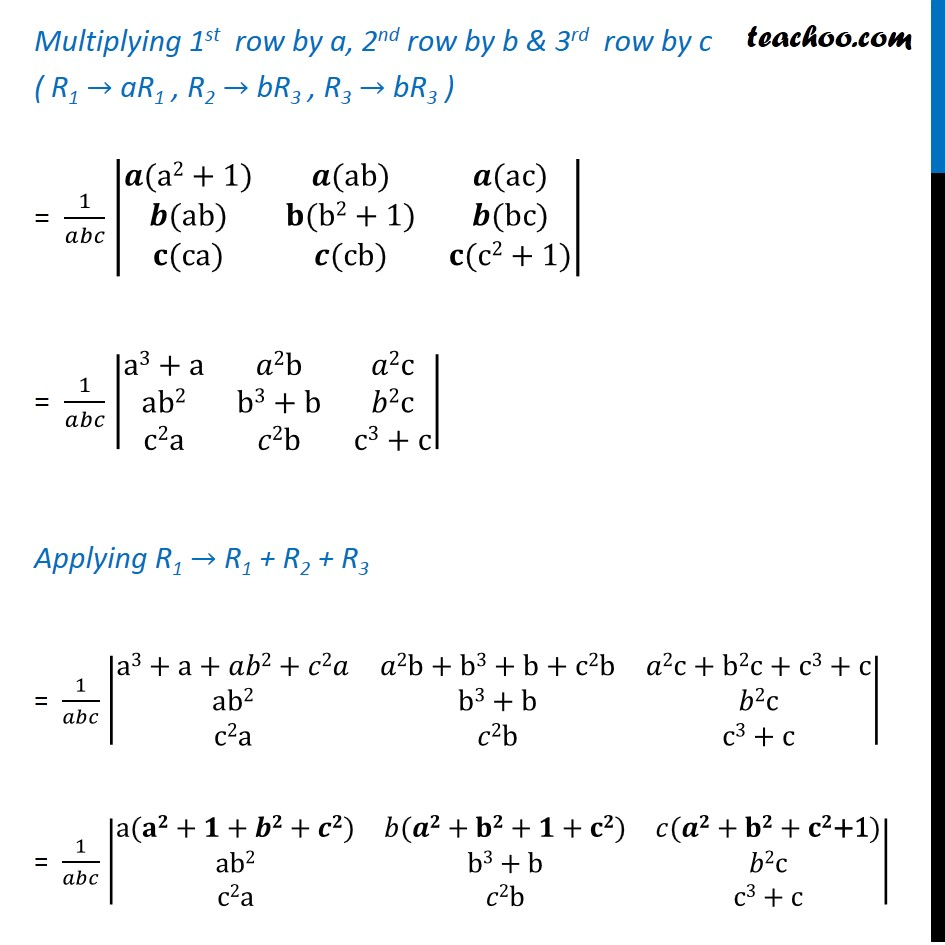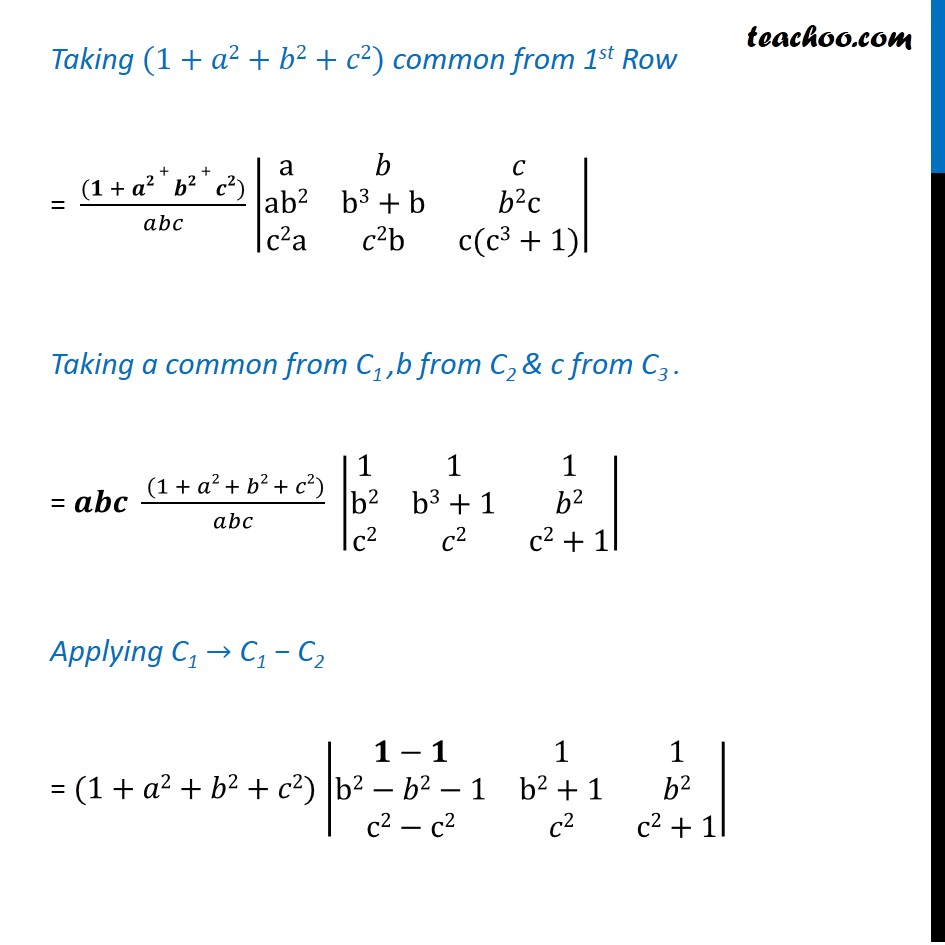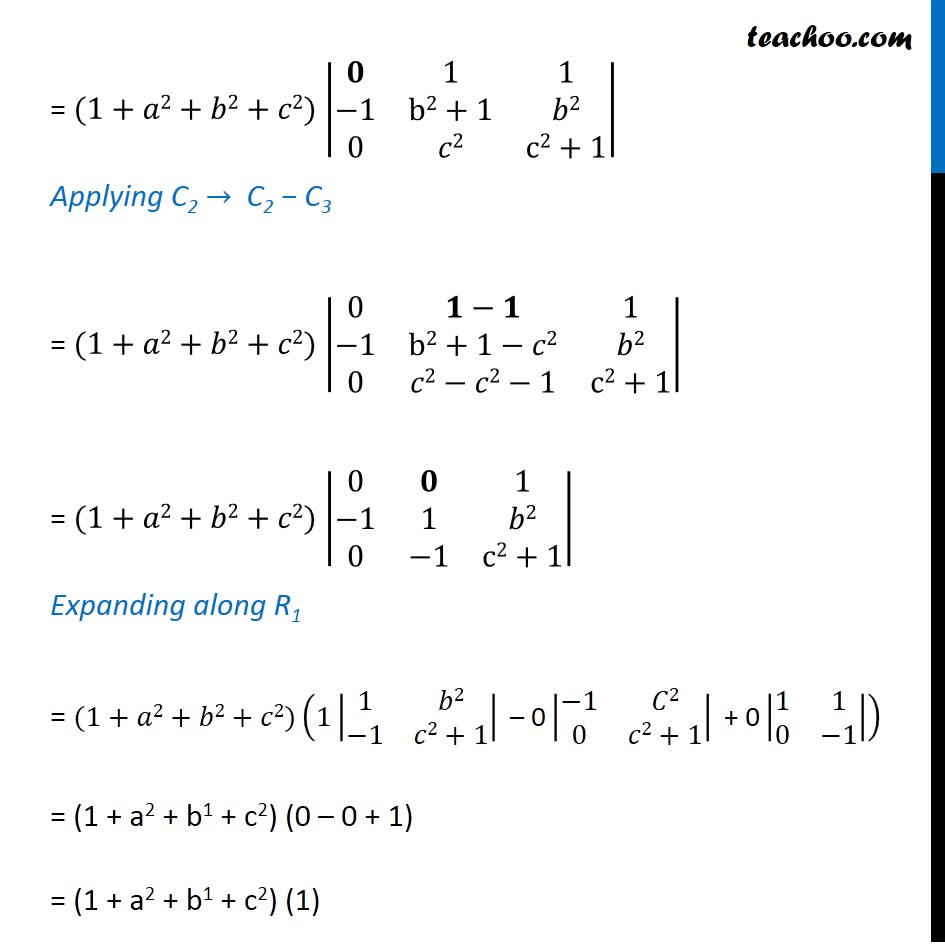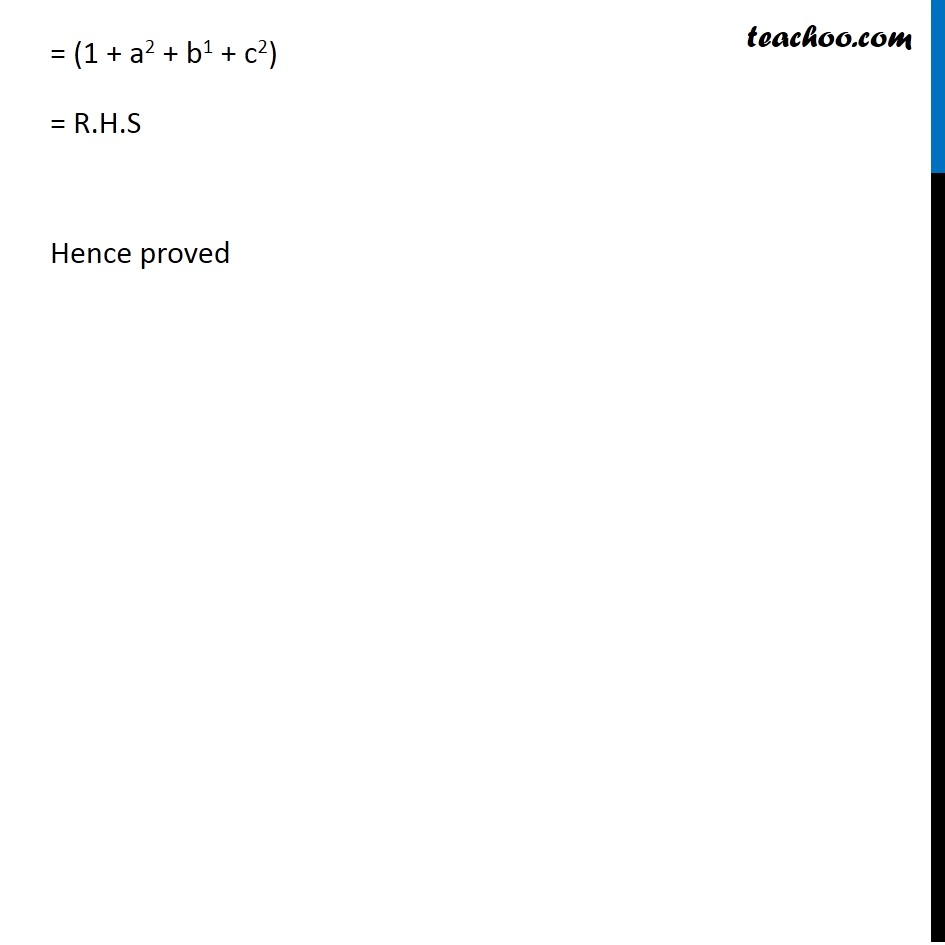Get live Maths 1-on-1 Classs - Class 6 to 12

### Transcript

Ex 4.2, 14 By using properties of determinants, show that: |■8(a2+1&ab&[email protected]&b2+1&[email protected]&cb&c2+1)| = 1 + a2 + b2 + c2 Solving L.H.S |■8(a2+1&ab&[email protected]&b2+1&[email protected]&cb&c2+1)| Multiplying & Dividing by abc = 𝒂𝒃𝒄/𝒂𝒃𝒄 |■8(a2+1&ab&[email protected]&b2+1&[email protected]&cb&c2+1)| Multiplying 1st row by a, 2nd row by b & 3rd row by c ( R1 → aR1 , R2 → bR3 , R3 → bR3 ) = 1/𝑎𝑏𝑐 |■8(𝒂(a2+1)&𝒂(ab)&𝒂(ac)@𝒃(ab)&𝐛(b2+1)&𝒃(bc)@𝐜(ca)&𝒄(cb)&𝐜(c2+1))| = 1/𝑎𝑏𝑐 |■8(a3+a&𝑎2b&𝑎[email protected]&b3+b&𝑏[email protected]&𝑐2b&c3+c)| Applying R1 → R1 + R2 + R3 = 1/𝑎𝑏𝑐 |■8(a3+a+𝑎𝑏2+𝑐2𝑎&𝑎2b+b3+b+c2b&𝑎[email protected]&b3+b&𝑏[email protected]&𝑐2b&c3+c)| = 1/𝑎𝑏𝑐 |■8(a(𝐚𝟐+𝟏+𝒃𝟐+𝒄𝟐)&𝑏(𝒂𝟐+𝐛𝟐+𝟏+𝐜𝟐)&𝑐(𝒂𝟐+𝐛𝟐+𝐜𝟐"+1" )@ab2&b3+b&𝑏[email protected]&𝑐2b&c3+c)| Taking (1+𝑎2+𝑏2+𝑐2) common from 1st Row = ((𝟏 + 𝒂𝟐 + 𝒃𝟐 + 𝒄𝟐))/𝑎𝑏𝑐 |■8(a&𝑏&𝑐@ab2&b3+b&𝑏[email protected]&𝑐2b&c(c3+1))| Taking a common from C1 ,b from C2 & c from C3 . = 𝒂𝒃𝒄 ( (1 + 𝑎2 + 𝑏2 + 𝑐2))/𝑎𝑏𝑐 |■8(1&1&[email protected]&b3+1&𝑏[email protected]&𝑐2&c2+1)| Applying C1 → C1 − C2 = (1+𝑎2+𝑏2+𝑐2) |■8(𝟏−𝟏&1&[email protected]−𝑏2−1&b2+1&𝑏[email protected]−c2&𝑐2&c2+1)| = (1+𝑎2+𝑏2+𝑐2) |■8(𝟎&1&[email protected]−1&b2+1&𝑏[email protected]&𝑐2&c2+1)| Applying C2 → C2 − C3 = (1+𝑎2+𝑏2+𝑐2) |■8(0&𝟏−𝟏&[email protected]−1&b2+1−𝑐2&𝑏[email protected]&𝑐2−𝑐2−1&c2+1)| = (1+𝑎2+𝑏2+𝑐2) |■8(0&𝟎&[email protected]−1&1&𝑏[email protected]&−1&c2+1)| Expanding along R1 = (1+𝑎2+𝑏2+𝑐2)(1|■8(1&𝑏[email protected]−1&𝑐2+1)|" – 0" |■8(−1&𝐶[email protected]&𝑐2+1)|" + 0" |■8(1&[email protected]&−1)|) = (1 + a2 + b1 + c2) (0 – 0 + 1) = (1 + a2 + b1 + c2) (1) = (1 + a2 + b1 + c2) = R.H.S Hence proved